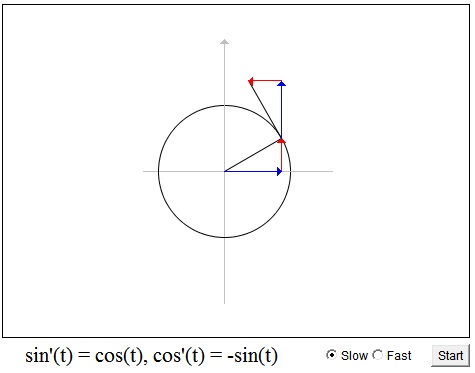# Derivatives of Sine and Cosine

Velocity is tangent to the line of motion. If a point rotates around a circle, its velocity is perpendicular to its radius-vector. Assume the point rotates around a unit circle. Then the radius-vector of the point is given by its projections on the two axes, which are cos(t) and sin(t):

 (1) r = (cos(t), sin(t)).

The derivative of (1)

 (2) r' = (cos'(t), sin'(t)).

is exactly the velocity of the point. If it to be perpendicular to r, we must have

 (3) cos(t)·cos'(t) + sin(t)·sin'(t) = 0.

This of course could be derived by differentiating the famous trigonometric form of the Pythagorean theorem

 (4) cos²(t) + sin²(t) = 1.

The applet below gives a geometric meaning of (3). Note how there are always two equal right triangles, with one rotated 90° with respect to the other. Note also how the direction of this rotation changes four times per revolution. Lastly, pay attention to the relative behavior of cos(t), the blue arrow, and the sin(t), the red arrow. sin(t) grows when cos(t) is positive, which happens in the right half plane. sin(t) decreases in the left half plane when cos(t) is negative. This suggests sin'(t) = cos(t). For cos'(t) the situation is different. For example, in the upper half plane, where sin(t) is positive, cos(t) decreases. It increases in the lower half plane where sin(t) is negative. This suggests cos'(t) = -sin(t).

### If you are reading this, your browser is not set to run Java applets. Try IE11 or Safari and declare the site https://www.cut-the-knot.org as trusted in the Java setup.What if applet does not run?

The above serves an additional purpose: it illustrates Euler's formula

eit = cos(t) + i·sin(t).

Indeed, if we knew up front that the complex valued function f(t) = eit could be differentiated with respect to t as if it were a real valued function, i.e. as in (eat)' = aeat, we would write

(eit)' = ieit.

But then from the geometric meaning of multiplication by i, the direcvative of eit would be perpendicular to eit and would have exactly same constant modulus as the function itself. Must not it be tangent to the curve traced by eit? See [Zeitz, p. 142] and [Needham].

(eit)' = -sin(t) + i·cos(t).

How fitting!

### References

1. T. Needham, Visual Complex Analysis, Oxford University Press; Reprint edition (February 18, 1999)
2. P. Zeitz, The Art and Craft of Problem Solving, John Wiley & Sons, 1999### Trigonometry